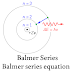## Balmer's lines Equation.Balmer's lines are used in nuclear physics. They are caused in the spectral lines of the emission of the hydrogen atom. Waveband shows an available wave with a certain length. This length reflects the visible spectrum and is different from the color indicated on the tape. The series of the hydrogen atom affecting the emission spectrum are six in number. In red Landa is λ= 6562.8 when we have H- alpha, blue color- λ= 4,861, while violet light can be observed three wavelengths to color and its intensity λ=y 4340.5, 4101.7, 3970.1, 3889.1. The wavelength is 400nm is in ultraviolet specter. This available light reflection and colors available on the spectral line can be monitored and only when there is the movement of electrons from level 3 to level 2 layers of layers.
The lines are defined by the following equations:

Where:
1. λ is the wavelength ;
2. R H is a Constant of Rydberg, which is non-changeable and is approximately = 109.689 cm -1 ;
3. N is the number of general layers and can be written not only with numbers and with alphabet symbols :
4. K, L, M, O, P, Q into electron diagrams;
5. The formula of Balmer’s series:

### Balmer’s series.

Balmer’s series is the visible spectrum. They all comprise the number of the layer n 1 = 2 and layer respectively, which is denoted n 2 correspond to levels = 3, 4, 5 and so on. Balmer's series may be calculated by the following formula:

1. λ is the wavelength ;
2. R H is a Constant of Rydberg, which is non-changeable and is approximate = 109.689 cm-1 ;
3. Where n2  > n1 ;

### This formula can be calculated.

All spectral lines with the participation of hydrogen. By equation, Balmer can determine the level of emission absorption and absorption compared to lines that appear spectrally.

Where :
B is a constant Balmar 3.64 x 10 -7 nm; where n and m are in the following n&gt; m, m~ 2,
1. λ is the wavelength ;
2. The importance of the equation is the following:
3. - Through it may be set at a surface temperature;
4. - Mr. catchment and determination include surface gravity and composition;
The physicist Rydberg the first place Balmеr’s equation for the passage of hydrogen. The
equilibrium of opportunity to calculate the series of Balmer include Ridberg‘s constant
hydrogen. So reformatted mathematical formula may appear in the following way:

Where:
1. λ is the wavelength of absorption of the emitted light
2. R H is the Rydberg constant for hydrogen. In the equilibrium, we can see that the Rydberg constant
3. is equivalent of or means that it is 4 divide of constant of Balmer.
4. And the B is constant of the value of 3.645 x 10 -7m;
5. The n here is the more value of m; n> m

#### Balmer‘s lines can appear as a level of absorption or emission band in the spectrum.

These types were dependent on the environment and nature in general. In spectral hydrogen epsilon lines sometimes calcium may be confused with another chemical
element, such as hydrogen. This can happen when he is in an ionized form. Only then the
absorption line is a shift from Level 7 to Level 2. When hydrogen-zeta potential for confusion helium hydrogen is great because of the level of 8 passes to 2. In exploration, too stars in astronomy it is possible to observe this because the stars are much greater temperature and helium in large quantity attitude.

## Author: N. Petkova Location: Bulgaria

Balmer Series | Balmer series equation, balmer series definition |Reviewed by Genuine Chemistry on February 02, 2020 Rating: 5